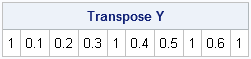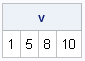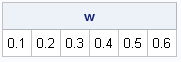It is common to want to extract the lower or upper triangular elements of a matrix. For example, if you have a correlation matrix, the lower triangular elements are the nontrivial correlations between variables in your data. As I've written before, you can use the VECH function to extract the lower triangular elements from a matrix:

```proc iml; /* 1. define a correlation matrix */ R = {1.0 0.1 0.2 0.3, 0.1 1.0 0.4 0.5, 0.2 0.4 1.0 0.6, 0.3 0.5 0.6 1.0 };   Y = vech(R); /* extract lower triangular elements, including the diagonal */ print (Y`)[label="Transpose Y"];```The elements are extracted in column-major order. Of course, for a symmetric matrix (such as a correlation matrix) the lower triangular elements in column-major order are the same as the upper triangular elements in row-major order.

However, each diagonal element of a correlation matrix is 1, so there is no need to store these values. How can you extract only the nontrivial correlation coefficients?

There is no built-in function in the SAS/IML runtime library that extracts the elements of R that are strictly below the diagonal, but it is easy enough to remove the diagonal elements. In the 4x4 example, notice that if you can remove the first, fifth, eighth, and tenth elements of Y, you will obtain the elements of R that are strictly below the diagonal.

Although the numbers 1, 5, 8, and 10 don't seem to exhibit a pattern, the intervals between these numbers form a decreasing arithmetic sequence: 4, 3, and 2. A moment's thought should convince you that for a p x p matrix, the following statements produce the indices of the elements of Y that you need to remove:

```/* 2. Generate the indices that correspond to the diagonal elements */ p = ncol(R); k = p:2; v = cusum( 1 || k ); print v;```The CUSUM function computes the cumulative sums of the sequence {1 4 3 2}. Thus the vector v contains the indices that correspond to the diagonal elements of R. To remove these values from the vector Y you can use the REMOVE function, as follows:

```/* 3. Use the REMOVE function to exclude the indices */ w = remove(Y, v); print w;```Success! The vector w contains the elements of R that are strictly below the diagonal. These are the nontrivial correlations in the correlation matrix.

If you want to do this operation more than once, it is useful to encapsulate the technique into a user-defined function, as follows:

```/* 4. User-defined function to extract elements below the diagonal */ start StrictLowerTriangular(X); v = cusum( 1 || (ncol(X):2) ); return( remove(vech(X), v) ); finish;   w = StrictLowerTriangular(R); /* test the function */ print w;```

The output of the function is the same as was shown previously.

Of course, the same trick works for extracting the strictly upper triangular portion of a matrix: just pass the transpose of the matrix to the StrictLowerTriangular function.

In summary, this technique uses three functions (VECH, CUSUM, and REMOVE) to write a function that extracts the lower triangular portion of a matrix. These three functions are not ones that I use every day, but as this example shows, they can be useful functions to know.

ShareDistinguished Researcher in Computational Statistics

Rick Wicklin, PhD, is a distinguished researcher in computational statistics at SAS and is a principal developer of SAS/IML software. His areas of expertise include computational statistics, simulation, statistical graphics, and modern methods in statistical data analysis. Rick is author of the books Statistical Programming with SAS/IML Software and Simulating Data with SAS.

1.Hi Rick,
Another application for the "cusum( 1 || " construct. I like it!

How about something along the lines of:

```start SLT(X); i=repeat(1:nrow(X),nrow(X),1); return( X[ loc(i<i `) ] ); finish;```

which returns the same in row major order? Conceptually it's simpler, but unfortunately it is not as quick.

•Yes, that's another approach. It uses the "row=col" trick that I wrote about in the article "Defining Banded Matrices." I discuss the efficiency of that method in the article.

2.This was a huge help! Thanks.

Follow up question: Now that I have the lower triangle elements, how do I get the labels for those elements? For example, if I want to report the correlations in columnar form, I need to label which two variables the correlations represent, like this:

Variable1 Variable2 Corr
X1 X2 .55
X1 X2 .44
X2 X3 .33
...

I now have the Corr column. How can I get the others?

Thanks,
Jeff

•Take a look at my article on pairwise correlations, which shows how to create labels for the pairs of variables. In fact, you might prefer the method in that article over the one in this article. If not, I can show how to modify this example.